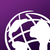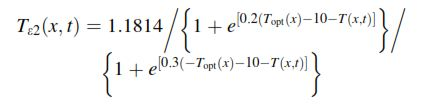# ArcPy Script output not matching with output generated from Arc GIS raster calculator

648
2
11-25-2016 07:19 AMOccasional Contributor III

Xander Bakker I am running into a problem of the mathematical expression in raster calculation. Earlier we had worked for writing the below code. According to existing mathematical function in the script, if raster cell value will be

Earlier we had worked for writing the below code. According to existing mathematical function in the script, if the raster cell value (Mean raster in script ) will be increase or cross the threshold value (23 mentioned in script) , output raster cell should be decreased . But with this code, I am getting reverse output which is not desirable. I have checked the same mathematical equation in Arc GIS raster calculator and Excel also, both results are same.  But Output  generated from below script is not matching with Excel and Arc GIS raster calculator's result . Kindly provide your guidance to solve the issue .

Below I have attached the link for raster data, which I am working on. Due to size limit here, I just uploaded on dropbox.

I am using Arc GIS 10.3 and script running on PyScripter

In below  equation Topt = 23 which is constant value (in our script) and T = Monthly mean raster data which is changed monthly.

Data link : Dropbox - TEST_TSCALAR1.rarScript copied from Arc GIS raster result :

``arcpy.gp.RasterCalculator_sa("""(1.185 / (1 + Exp(0.2 * (23 - 10 - "Mean_Temp_010.tif"))) / (1 + Exp(0.3 * ( - 23 - 10  + "Mean_Temp_010.tif")))) * 0.99""", "D:/NPP_GUJARAT/WEATHER_DATA/2012/T_SCALAR_2012C/T_SCALAR_010A.tif")‍‍``

ArcPy Script,

``````import arcpy, os, calendar
from arcpy.sa import *
from arcpy import env
arcpy.env.overwriteOutput = True
arcpy.CheckOutExtension("Spatial")
topWorkspace = r'D:\TEST_TSCALAR'
ws_out = r'D:\TEST_TSCALAR\T_SCALAR_OUT' ### Output folder!!!
arcpy.env.workspace = topWorkspace

# Get dict of months and month-number (i.e. January = 001, March = 003 etc.)
months = {calendar.month_name[i].upper(): str(i).zfill(3) for i in range(1, 13)}

# Step through list of all folders
for folderPath in arcpy.ListWorkspaces():
baseName = os.path.basename(folderPath).upper()
if baseName in months: # Test that subfolder is a month name
monthNumber = months[baseName] # Get month-number for use in output filename
arcpy.env.workspace = folderPath

# Raster1 take Mean_Temp raster from all month folder(e.g for january: Mean_temp_001.tif) and Raster 2 is constant month of october raster
rasterList1 = arcpy.ListRasters(r'Mean_Temp*.tif')

print rasterList1
for ras_name in rasterList1:
ras_mean = arcpy.Raster(os.path.join(folderPath, ras_name))

# I devided the above equation into two part
# TScalar2 (Exp = exponential)
ras_scal2 = 1.1814 / (1 + Exp(0.2 * (23 - 10 - ras_mean)))

# TScalar3
ras_scal3 = (1 + Exp(0.3 * (- 23  - 10 - ras_mean)))

# Multiply TScalar1 and TScalar2
ras_scal = 0.99 * (ras_scal2 / ras_scal3)

# Save the output
outRasterName = os.path.join(ws_out, "T_SCALAR_{}.tif".format(monthNumber))
#print outRasterName
ras_scal.save(outRasterName)

#print done
print 'done'
‍‍‍‍‍‍‍‍‍‍‍‍‍‍‍‍‍‍‍‍‍‍‍‍‍‍‍‍‍‍‍‍‍‍‍‍‍‍‍‍‍‍‍‍‍‍‍‍‍‍‍‍‍‍‍‍‍‍‍‍‍‍‍‍‍‍‍‍‍‍‍‍‍‍‍‍‍‍‍‍‍‍‍‍‍‍‍‍‍‍‍‍‍‍‍‍‍‍‍‍‍‍‍‍‍‍‍‍‍‍‍‍‍‍‍‍‍‍‍‍‍‍‍‍‍‍‍‍‍‍‍‍‍‍‍‍‍``````
Tags (2)
1 Solution

Accepted SolutionsbyMVP Esteemed Contributor

In your first code snippet, the last part of your equation is  -meantemp, you have +meantemp which disagrees with the equation symbols... Which is it? A minus here, a plus there, will make a difference

2 RepliesbyMVP Esteemed Contributor

In your first code snippet, the last part of your equation is  -meantemp, you have +meantemp which disagrees with the equation symbols... Which is it? A minus here, a plus there, will make a differenceOccasional Contributor III

Dan Patterson‌, Thank you very much. Exactly you hit the source of error. Sorry for

Xander Bakker  Sorry for the inconvenience.

Main reason for error is:

1. During script writing, i followed the wrong equation (Snapshot) which is published by co-research author.

2. During Arc GIS raster calculator i followed the right equation which is published by main research author.

I figured out the error with the snapshot equation after Dan Patterson pointed the minus and plus difference. And i checked the main research paper also from where  co-author has taken the equation.

And which equation i used in Arc GIS raster calculator and Excel sheet that is  correct, that's why i was continuous getting the wrong result.

Now i am getting the accurate result from both of programs.

Once again thank you very much to all.